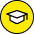You searched for - dummies

Showing 41 Search Results for Amber kuang

There are no results for available. Check the spelling and try again, or check the most popular results from this topic.

Search Result
Sort By

41 results found.

•In Geometry

March 27,2016

### 1,001 Geometry Practice Problems For Dummies Cheat

Geometry is full of formulas, properties, and theorems. You can become successful in geometry by remembering the most important ones and learning how...

•In Geometry

March 26,2016

### Circle Basics for Geometry Problems

To solve geometry problems about circles, you will need to know the following circle theorems involving tangents, secants, and chords. These theorems...

•In Geometry

March 26,2016

### Coordinate Geometry Formulas

Coordinate geometry is the study of geometric figures graphed on a coordinate plane. The slope formula can be used to determine whether lines are para...

•In Geometry

March 26,2016

### Geometry Practice Problems with Triangles and Poly

A polygon is a geometric figure that has at least three sides. The triangle is the most basic polygon. You will find the following formulas and proper...

•In Geometry

March 26,2016

### Formulas for Geometric Solids Problems

Many formulas are associated with the study of three-dimensional shapes in geometry. Here, you find formulas for calculating the volume, surface area,...

•In Geometry

March 26,2016

### Transformation Rules for Geometry Problems

In coordinate geometry problems, there are special rules for certain types of transformations. To determine the image point when performing reflection...

•In Geometry

March 26,2016

### Angles That Form Linear Pairs—Practice Geome

Angles that form a linear pair combine to form a straight angle. (A straight angle measures 180 degrees.) The following practice questions ask you to...

•In Geometry

March 26,2016

### Area of Regular Polygons — Practice Geometry

If you are asked to find the area of a regular polygon, you can do so by using a formula that includes the perimeter of the polygon and a measurement...